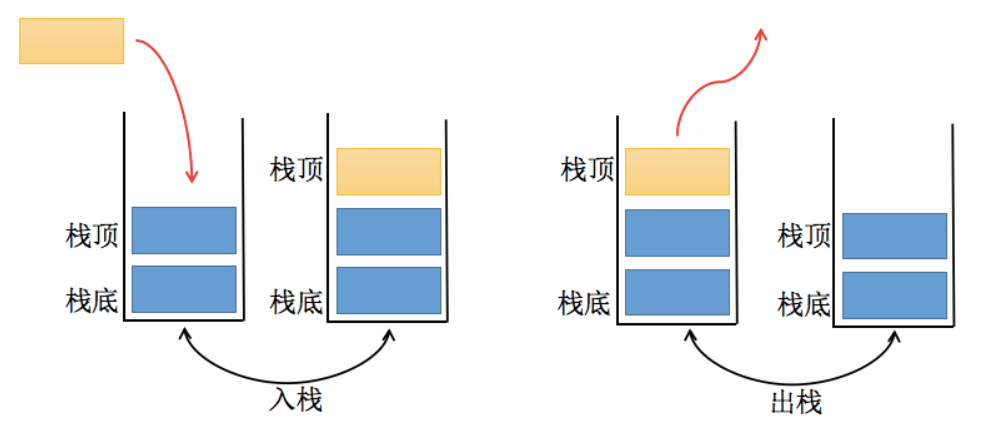# # 栈

TIP

## # 基本特点

• 受限表现：仅允许一端插入和删除，这端称为栈顶，另一端称为栈底
• LIFO：最后进入的元素，最先弹出栈空间，类似自动餐托盘，最后放上去最先被拿走
• 插入元素称为：进栈、入栈、压栈，它是把新元素放到栈顶元素上面，使之成为新的栈顶元素
• 删除元素称为：出栈、退栈，他是把栈顶元素删除掉，使其相邻的元素称为新的栈顶元素

## # 结构图例## # 常用操作

• push(element)：添加一个新元素到栈顶位置
• pop()：移除栈顶的元素，同时返回被移除的元素
• peek()：返回栈顶的元素，不对栈做任何修改（该方法不会移除栈顶的元素，仅仅返回它）
• isEmpty()：如果栈里没有任何元素就返回 true，否则返回 false
• size()：返回栈里的元素个数。这个方法和数组的 length 属性类似
• toString()：将栈结构的内容以字符串的形式返回

## # 代码实现

``````// 栈类
function Stack() {
// 栈中的属性
this.items = []

/* 栈相关的方法 */
// 1.push(): 压栈操作
Stack.prototype.push = function(element) {
this.items.push(element)
}

// 2.pop()：从栈中取出元素
Stack.prototype.pop = function() {
return this.items.pop()
}

// 3.peek()：返回栈顶元素
Stack.prototype.peek = function() {
return this.items[this.items.length - 1]
}

// 4.isEmpty()：判断栈中的元素是否为空
Stack.prototype.isEmpty = function() {
return this.items.length == 0
}

// 5.size()：获取栈中元素的个数
Stack.prototype.size = function() {
return this.items.length
}

// 6.toString()：以字符串形式输出栈内数据
Stack.prototype.toString = function() {
let resultString = ""
for (let i = 0; i < this.items.length; i++) {
resultString += this.items[i] + " "
}
return resultString
}
}

// 模拟面试题
var stack = new Stack()

stack.push(6)
stack.push(5)
stack.push(4)
stack.push(3)

console.log(stack.pop()) // 3
console.log(stack.peek()) // 4
console.log(stack.isEmpty()) // false
console.log(stack.size()) // 3
console.log(stack.toString()) // 6 5 4
``````

## # 程序应用

### # 函数调用栈

``````A(B(C(D())))

``````

### # 递归

``````自己调用自己

``````